Search IntMath
Close

450+ Math Lessons written by Math Professors and Teachers

5 Million+ Students Helped Each Year

1200+ Articles Written by Math Educators and Enthusiasts

Simplifying and Teaching Math for Over 23 Years

# IntMath Newsletter: Hexagon, units, primes, Queen

By Murray Bourne, 14 Dec 2018

14 Dec 2018

1. New on IntMath: CSS Animated Hexagon Wave
2. Units
3. Math in the news: Almost prime numbers
4. Brian May - astrophysicist
4. Math movies: Data portraits and fingers
5. Math puzzle: Divisibility
6. Final thought: It's up to us

## 0. Season's greetings

Here's wishing you all an enjoyable New Year break, no matter your faith. One of the most popular articles on the blog this time of year is:

The Twelve Days of Christmas - How Many Presents?

All the best to you for 2019!

## 1. New on IntMath

### Math Art in Code: CSS Animated Hexagon Wave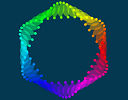Here's an animation made from pure CSS whose wave motion makes it look a bit like a jelly fish. Two triangles intersect to form a stylized hexagon.

## 2. Units

Two related stories about units came my way recently.

### (a) Calorie or calorie?

It seems there is more than one calorie. Which one should we use?

### (b) The kilogram is changing

The kilogram's definition, based on a metal cylinder, had a problem - it was losing mass. Time for a makeover.

## 3. Almost Prime numbers

We know primes have at most 2 factors, 1 and themselves:

2 = 1 × 2
3 = 1 × 3
5 = 1 × 5
7 = 1 × 7
...

Now consider these numbers:

4, 6, 9, 10, 14, 15, ...

Let's look at their prime factors:

4 = 2 × 2
6 = 2 × 3
9 = 3 × 3

10 = 2 × 5
14 = 2 × 7
15 = 3 × 5

Each of them has 2 prime factors. Mathematicians call these "almost prime numbers", and in this case "2-almost prime".

We can also have a set of "3-almost primes", with 3 prime factors each:

8 = 2 × 2 × 2
12 = 2 × 2 × 3
20 = 2 × 2 × 5
28 = 2 × 2 × 7
30 = 2 × 3 × 5
...

What's the point of these "almost primes"? Read more about them and see some of the ways they've been employed to solve some of the most challenging proofs in number theory (which are important in the fields of data encryption) at:

## 4. Brian May - astrophysicistdBrian May [Photo credit]

I recently enjoyed the Queen biopic Bohemian Rhapsody. I was reminded of the educational attainments of the bands' members (degrees in biology, electronics and graphic design), especially that of Brian May's.

May put his PhD studies on hold as the band gained in popularity, and returned to complete it in 2007, submitting it to Imperial College of Science, Technology and Medicine.

His PhD, A Survey of Radial Velocities in the Zodiacal Dust Cloud (PDF, 256pp) uses integral and differential calculus, and not suprisingly, trigonometry.

The zodiacal light, also called the "false dawn" is sunlight reflected by dust particles between the Sun and Earth close to sunrise or sunset.Credit: ESO/Y. Beletsky

Brian May was also a mathematics teacher for a time at Stockwell Manor School, Brixton, London.

## 5. Math Movies

### (a) Insightful human portraits made from data

I'm a big fan of data visualizations, and I believe it's a field that will provide a lot of employment in the foreseeable future.R. Luke DuBois creates fascinating portraits from data which help us to see ourselves in new ways.

### (b) How high can you count on your fingers? (Spoiler: much higher than 10)Children are discouraged from counting on their fingers, but they may be missing out on concrete understanding before they begin to think abstractly. This TED-Ed video explores how we can employ different number systems using our fingers.

## 6. Math puzzles

The puzzle in the last IntMath Newsletter asked about the sum of numbers in a box. Correct answers with sufficient explanation were submitted by Sue, Nathan, Georgios, Wesen, Douglas, Bandu, Francis and Tomas. Special mention to Eamon, who carefully showed his thinking processes.

### New math puzzle: Divisibility

Prove that 2n32n − 1 is divisible by 17 for all positive integral values of n.

You can leave your response here.

## 7. Final thought - it's up to us

I remember when scientists started talking about the potential for climate change a few decades ago. After repeated warnings, politicians in most countries have let us down, pandering to the "jobs and growth" mantra (which really means "the rich must become super rich, and the rest of us can go to hell"), and allowing the big companies to destroy the water, air, arable land and the forests.

It's going to be up to us - ordinary people - to make a difference. The politicians have had their chance and have failed.

"Do the difficult things while they are easy and do the great things while they are small." [Lao Tzu]

Until next time, enjoy whatever you learn.

1. Turab says:

Sir please give some example of area under the curve

2. Murray says:

@Turab: You'll find several examples (after the ads) on IntMath using this search: area under a curve

3. Ibrahim El Hage says: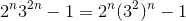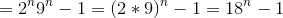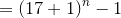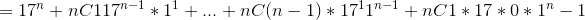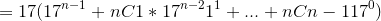4. Paul Holland says:

One can rewrite the expression as (18 to the power of n )-1 and do simple boring induction

5. Ajayshukla says:

Proving 2^n*3^(2n)-1 is divisible by 17 is equivalent to prove: 18^n = 1(mod 17).
We can prove this induction.
For n=1, the result is true.
Assume for n = k, 18^k = 1(mod 17)
Multiply 18 both side,
18^(k+1)= 18 = 1(mod 17)
So the statement is true for all natural number n.

6. Georgios Varelas says:

For n=1: (2^n)*(3^(2n)) – 1 = 2*9 – 1 = 18 – 1 = 17 = 17 * 1
Suppose it holds for n = n:
(2^n)*(3^(2n)) – 1 = 17*k, k=natural number = positive integral value
We will prove that it holds for n = n+1:
(2^(n+1))*(3^(2(n+1)))–1 = 17*m, m = natural number = positive integral value
(2^(n+1))*(3^(2(n+1))) – 1 – (2^n)*(3^(2n)) – 1 =
(2^n)*(3^(2n))*((2*9) – 1) =
(2^n)*(3^(2n))*17 = (17*k+1)*17 =>
(2^(n+1))*(3^(2(n+1))) – 1 – 17*k = (17*k+1)*17 =>
(2^(n+1))*(3^(2(n+1))) – 1 = (17*k+1)*17 + 17*k = (18*k+1)*17 = 17*m, m = 18*k+1
Therefore, it applies to any physical number n

7. Alex Green says:

Proof by mathematical Induction:

2^n 3^(2n) − 1 is divisible by 17, for n = 1
2^1 3^2 -1 = 17 which is divisible by 17

Assume that this true for n= k:

i.e. 2^k 3^(2k) − 1 = 17P where P is an positive integer:

RTP that 2^(k+1) 3^(2(k+1)) − 1 is also divisible by 17

2^(k+1) 3^(2(k+1)) − 1 = 2 2^k 3^2 3^(2k) - 1
=2^k 3^2k(2 3^2) -1
=(17P+1)(18) - 1 {from our assumption}
=17P(18) + 18 -1
=17P(18)+17
=17(18P+1)
=17Q, where Q = 18P+1

which is a multiple of 17

8. Elizabeth says:

Step 1: Prove true for n= 1
LHS = (2^1)(3^2x1) - 1
RHS = 17
therefore LHS = RHS
Step 2: Assume true for n=k
2^k * 3^2k = 17P (where P is an integer)
Step 3: Prove true for n= k+1
2^k+1 * 3^2(k+1) = 17Q (where Q is an integer)
LHS = 2(2^k) * 3^2(2^k)
LHS = 2(17P/3^2k) * 3x2(2^k) (by assumption)
= 34P/3^2k * 3*2^(2k)
34P *3 = 102P
17(6P) = 17Q
therefore true by induction

9. Russell says:

Hmmm...Dropbox seems to work better without html markup.

Solution on Dropbox

10. Charles Williams says:

The sum of digits when simplified when n is a positive integral is divisible by 17 and the digits add up to be divisible by 8 which the sum of the number 17 (1+7)

Sir,
The newsletter is very good. The proof asked in the puzzle can be attempted with "mathematical induction" theorem.

If i take the mathematical statement as
2^n.3^2n-1=17x_n where x_n is an integer. (1)

Proof:
For n=1, x_1 =1, an integer

If for n=k,
2^k.3^2k-1=17.x_k x_k is assumed to be integer. (2)

For n=k+1,
After substitution and rearrangement of expression (1)
x_(k+1)=18.x_k+1
which turns out to be integer as per our assumption at (2).

Hence, based on mathematical induction theorem, the statement is proven

12. Aidan Burke says:

proof by induction
true for n=1
(2^1)(3^2(1))-1=17
assume true for n=k

(2^k)(3^2k)-1=17(a)
prove true for n=k+1

(2^(k+1))(3^2(k+1))-1
∴(2)(9)((2^k)(3^2k)-1)+17
∴18((2^k)(3^2k)-1)+17
∴17(18(a))+17
∴17(18(a)+1) which is divisible by 17

true for 1 therefore true for 2 therefore true for 3 an for all positive integers

13. roger luther says:

This is proved using proof by induction in two lines!

14. Bandu says:

We use induction theory to prove that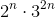is divisible by 17.

Let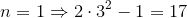.

Therefore,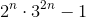is divisible by 17 when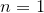.

Now, let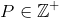and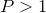.

Assume that,is divisible by 17.

Now consider,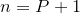.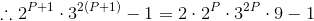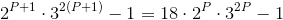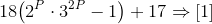Now consider,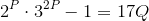where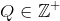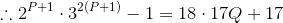from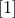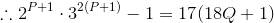is divisible by 17.

Hence,is divisible by 17.

15. Vijay Singh says:

(2*9)^n-1=(18)^n-1
Now 18^n can be written as (1+17)^n =C0+ C1(17) + C2(17)^2 + C3(17)^3 + so on up-to Cn(17)^n
Therefore
(18)^n-1=C1(17) + C2(17)^2 + C3(17)^3 + so on up-to Cn(17)^n
Taking 17 common from the whole tern
(18)^n-1=17α
Which shows that it is divisible by 17

16. Charles Witschorik says:

I have a proof by mathematical induction that I can send you as a pdf if you want more detail. The key statements in the inductive step follow,

2^(k+1)∙ 3^2(k+1) -1
=2^k∙2∙3^2k∙3^2-1
=((2^k 3^2k-1)+1)∙2∙3^2-1
=((17∙l)+1)∙18-1 -- inductive hypothesis; l an integer
=17∙l∙18+18-1
=17∙l∙18+17
=17∙(l∙18+1)
=17∙l'
l' =(l∙18+1), l' a positive integer.

17. TUGUME JONAN says:

Well. To be divisible by any number means your a multiple of that very number.
So (2^n)(3^2n)-1 to be divisible by 17 for all positive integrals of n.

Let's check whether it holds true for n=1.
(2^n)(3^2(n))-1=(2^1)(3^2(1))-1
=2(9)-1
=17 which is a multiple of 17.

Let's check whether it holds true for n=2.
(2^2)(3^2(2))-1=(4)(3^4)-1
=4(81)-1
=324-1
=323 which is a multiple of 17.
Since it holds for n=1,2..., it should hold for n=k.

Let's check whether it holds for n=(k+1)
(2^n)(3^2n)-1= (2^(k+1))(3^2(k+1))-1
=(2^k)(2^1)(3^2k)(3^2)-1
=(2^k)(3^2k)2(9)-1
=[(2^k)(3^2k)]18-1
Since (2^k)(3^2k) divides 17, then it also holds for n=(k+1)

18. Kevin Dudeja says:

The above equation becomes 18^n - 1

Method #1
The following can be seen of the form a^n - b^n which factors out (18-1)
Method #2
18^n - 1 can be written as (17-1)^n - 1
This always factors out 17 when the brackets are opened.

19. Tomas Garza says:

Use mathematical induction. Denote by p(n) the expression 2^n 3^(2n) - 1. p(1) equals 17, which is divisible by 17. Now, for any n, 2^n 3^(2n) = 18^n . Assume that, for a given n, p(n) is true, i.e., 18^n -1 is divisible by 17. Then, since p(n + 1) = 18^(n+1) - 1, this can be written as 18 *18^n - 1 = 18 (18^n - 1) + 18 - 1 = 18 (18^n -1) + 17 (after adding and subtracting 18). I.e., p(n + 1) = 18 (18^n -1) + 17 = 18 p(n) + 17.
The first term in the last expression is divisible by 17, since p(n) is too, while the second is also divisible by 17. The sum of two terms, each of which is divisible by 17, is also divisible by 17. This completes the proof.

20. Gerard Grufferty says:

Induction seems the way out.
(2^n).(3^2n) - 1 = 18^n - 1

Let P(n) be the proposition that 17 divides 18^n - 1

Then for P(1): 17 divides 18^1 - 1 = 17 and then (P(1)is true.

Assume P(k): i.e. that 17 divides 18^k - 1

Now consider P(k+1):
18^(k + 1) -1
= 18^(k + 1) - 18^k + 18^k -1
= 18^k(18 - 1) + (18^k -1)
= 18^k(17) + (18^k -1)
Now 17 divides 18^k(17) and 17 divides (18^k -1) by the assumption.
So 17 divides 18^(k + 1) -1
Then P(k) implies P(k+1)

So P(1) implies P(2)
and P(2) implies P(3)
and P(3) implies P(4)
and so on for all natural numbers by mathematical induction.

OR
Maybe using the series 1 + 18 + 18^2 = 18^3 + . . . . . = 18^(n-1)
= (1(18^n - 1))÷ (18 -1) using the sum to n terms of a geometric series.
It is clear that the series 1 + 18 + 18^2 = 18^3 + . . . . . = 18^(n-1) is an natural number.
and this series equals (1(18^n - 1))÷ (18 -1) and must be a natural number.

21. Alfredo A;ejandro Bustos-Ramirez says:

to show that( 2^n*3^(2n)-1) is divisible by 17, we first do some algebra in order to factorize the polynomial:
(2^n*3^(2n)-1)= (2^n*(3^2)^n-1)=( (2*9)^n-1)= (18^n-1)
(18^n-1)= (18-1)*(18^(n-1) +18^(n-2) +18^(n-3)+ .....+18 + 1)= 17*(18^(n-1) +18^(n-2) +18^(n-3)+ .....+18 + 1); which is divisible by 17

### Comment Preview

HTML: You can use simple tags like <b>, <a href="...">, etc.

To enter math, you can can either:

1. Use simple calculator-like input in the following format (surround your math in backticks, or qq on tablet or phone):
a^2 = sqrt(b^2 + c^2)
(See more on ASCIIMath syntax); or
2. Use simple LaTeX in the following format. Surround your math with $$ and $$.
$$\int g dx = \sqrt{\frac{a}{b}}$$
(This is standard simple LaTeX.)

NOTE: You can mix both types of math entry in your comment.